# Concentration at equilibrium?

The formation constant* of [M(CN)_6]^(4– is 2.50 × 10^17, where M is a generic metal. A 0.150-mole quantity of $M {\left(N {O}_{3}\right)}^{2}$is added to a liter of 1.220M NaCN solution. What is the concentration of ${M}^{2 +}$ ions at equilibrium?

Answer 1

Warning! Long Answer. The equilibrium concentration of $\text{M"^"2+}$ ions is 8.23 × 10^"-16"color(white)(l)"mol/L".

#### Explanation:

The balanced equation is

"M"^"2+" + "6CN"^"-" ⇌ "M(CN)"_6^"4+"; K_text(f) = 2.50 × 10^17

We can set up an ICE table.

$\textcolor{w h i t e}{m m m m m m m l} \text{M"^"2+" + color(white)(ll)"6CN"^"-" ⇌ "M(CN)"_6^"4+}$
$\text{I/mol·L"^"-1} : \textcolor{w h i t e}{m l l} 0.150 \textcolor{w h i t e}{m m} 1.220 \textcolor{w h i t e}{m m m l l} 0$
$\text{C/mol·L"^"-1": color(white)(mm)"-"xcolor(white)(mmmll)"-6"xcolor(white)(mmml)"+} x$
$\text{E/mol·L"^"-1": color(white)(m)"0.150-"xcolor(white)(m)"1.220-6} x \textcolor{w h i t e}{m m l} x$

K_text(f) = (["M(CN)"_6^"4+"])/(["M"^"2+"]["CN"^"-"]^6) = x/(("0.150-"x)(1.220-6"x)^6) = 2.50 × 10^17

This result looks rather unpromising. We must solve a seventh-order polynomial.

However, the value of ${K}_{\textrm{f}}$ is so large that the reaction goes substantially to completion.

Thus, 0.150 mol of $\text{M"^"2+}$ will react with 0.900 mol of $\text{CN"^"-}$ and form 0.150 mol of $\text{M(CN)"_6^"4+}$ (with 0.300 mol of $\text{CN"^"-}$ unused).

We can approach the equilibrium from the other end. Let's assume that the reaction goes to completion and write the equilibrium in reverse.

$\text{M(CN)"_6^"4+" ⇌"M"^"2+" + "6CN"^"-}$

Then,

K = 1/K_text(f) = 1/(2.50 × 10^17) = 4.00× 10^"-18"

Our new ice table becomes

$\textcolor{w h i t e}{m m m m m m} \text{M(CN)"_6^"4+" ⇌ "M"^"2+" + "6CN"^"-}$
$\text{I/mol·L"^"-1} : \textcolor{w h i t e}{m l l} 0.150 \textcolor{w h i t e}{l l m m m} 0 \textcolor{w h i t e}{m m l} 0.300$
$\text{C/mol·L"^"-1": color(white)(mm)"-"xcolor(white)(mmmm)"+"xcolor(white)(mm)"+6} x$
$\text{E/mol·L"^"-1": color(white)(m)"0.150-"xcolor(white)(mmm)xcolor(white)(ml)"0.300+6} x$

K = (["M"^"2+"]["CN"^"-"]^6)/(["M(CN)"_6^"4+"]) = (x("0.300+6"x)^6)/("0.150-"x)= 4.00 × 10^"-18"

Check for negligibility

0.300/(4.00 × 10^"-18") = 7. 5 × 10^16 > 400. ∴ 6x ≪ 0.300 and x ≪ 0.150.

Then,

(x(0.300)^6)/0.150 = 4.00 × 10^"-18"

7.29 × 10^"-4"x = 0.150 × 4.00 × 10^"-18" = 6.00 × 10^"-19"

x = (6.00 × 10^"-19")/(7.29 × 10^"-4") = 8.23 × 10^"-16"

["M"^"2+"] = 8.23 × 10^"-16"color(white)(l)"mol/L"

Check:

K = ((8.23 × 10^"-16")(0.300 + 6 × 8.23 × 10^"-16")^6)/("0.150 -8.23 × 10"^"-16") = ((8.23 × 10^"-16")(0.300)^6)/0.150 = 4.00 × 10^"-18"#

It checks!

#### Earn Coin

Coins can be redeemed for fabulous gifts.

Similar Homework Help Questions
• ### The formation constant* of [M(CN) 6 ]4− is 2.50 × 10 17 , where M is...

The formation constant* of [M(CN) 6 ]4− is 2.50 × 10 17 , where M is a generic metal. A 0.140 mole quantity of M(NO3)2 is added to a liter of 1.210 M NaCN solution. What is the concentration of M2+ ions at equilibrium?

• ### The formation constant* of [M(CN)6]4– is 2.50 × 1017, where M is a generic metal. A...

The formation constant* of [M(CN)6]4– is 2.50 × 1017, where M is a generic metal. A 0.170-mole quantity of M(NO3)2 is added to a liter of 1.41 M NaCN solution. What is the concentration of M2 ions at equilibrium?

• ### The formation constant of M(CN)614-is 2.50 x 10'7, where M is a generic metal. A 0.130...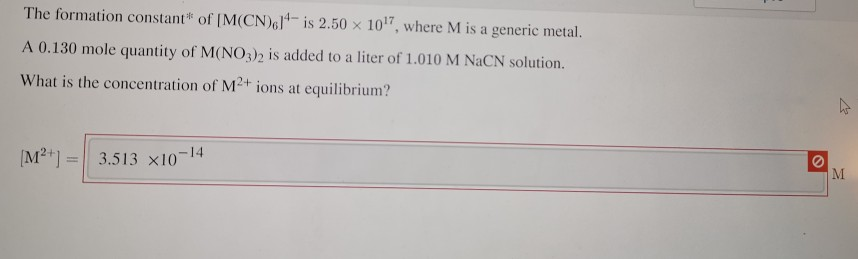The formation constant of M(CN)614-is 2.50 x 10'7, where M is a generic metal. A 0.130 mole quantity of M(NO3)2 is added to a liter of 1.010 M NaCN solution. What is the concentration of M2+ ions at equilibrium? [M²+] = 3.513 x10-14

• ### The formation constant* of [M(CN) 4 ]2− is 7.70×1016 , where M is a generic metal....

The formation constant* of [M(CN) 4 ]2− is 7.70×1016 , where M is a generic metal. A 0.130 mole quantity of M(NO3)2 is added to a liter of 0.870 M NaCN solution. What is the concentration of M2+ ions at equilibrium?

• ### The formation constant* of [M(CN)2]– is 5.30 × 1018, where M is a generic metal. A...

The formation constant* of [M(CN)2]– is 5.30 × 1018, where M is a generic metal. A 0.170-mole quantity of M(NO3) is added to a liter of 0.540 M NaCN solution. What is the concentration of M ions at equilibrium?

• ### The formation constant* of [M(CN)2]-is 5.30 x 1018, where M is a generic metal. A 0.170...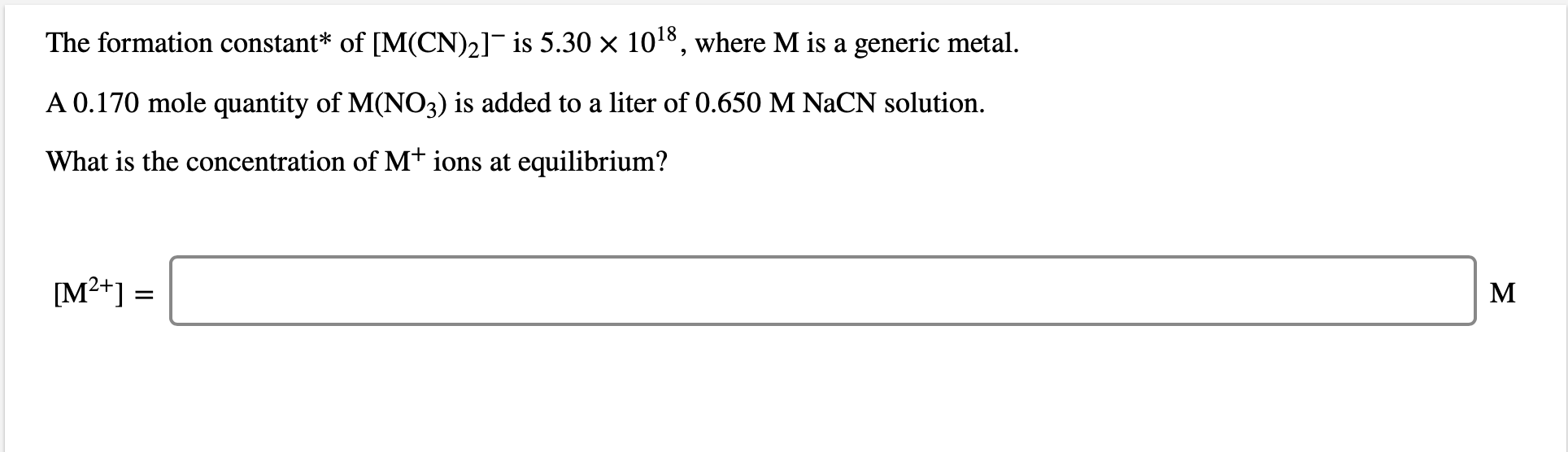The formation constant* of [M(CN)2]-is 5.30 x 1018, where M is a generic metal. A 0.170 mole quantity of M(NO3) is added to a liter of 0.650 M NaCN solution. What is the concentration of M+ ions at equilibrium? [M2+] =

• ### The formation constant of M(CN),1'-is 2.50 x 101, where M is a generic metal A 0.130...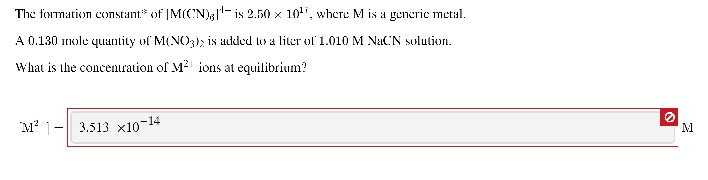The formation constant of M(CN),1'-is 2.50 x 101, where M is a generic metal A 0.130 wole quantity of M(NO3)> is alled to a liter of' 1.010 M NaCN solution. What is the concentration of Mº ions at equilibrium? M? 1- 3.513 x10-14

• ### can someone please help me answer these, for some reason theh are both wrong and i cant get them right, will rate y...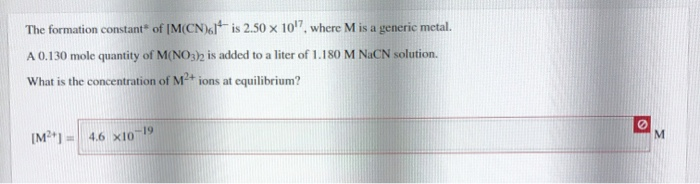can someone please help me answer these, for some reason theh are both wrong and i cant get them right, will rate you good if you can help get both correct answers! thanks The formation constant of M(CN)61 is 2.50 x 10'7, where M is a generic metal. A 0.130 mole quantity of M(NO3) is added to a liter of 1.180 M NaCN solution. What is the concentration of M2+ ions at equilibrium? [M ] = 4.6 x10-19 The generic...

• ### A 0.180 mole quantity of NiCl, is added to a liter of 1.20 M NH, solution....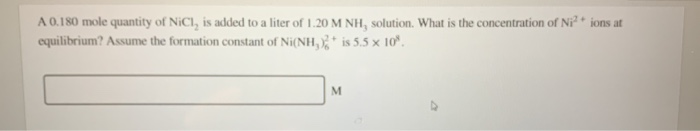A 0.180 mole quantity of NiCl, is added to a liter of 1.20 M NH, solution. What is the concentration of Ni?+ ions at equilibrium? Assume the formation constant of Ni(NH)2+ is 5.5 x 10

• ### A 0.170 mole quantity of NiCl, is added to a liter of 1.20 M NH, solution....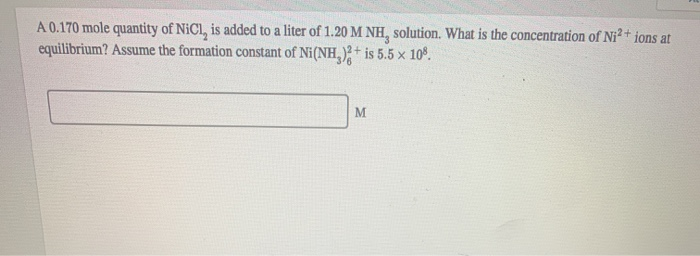A 0.170 mole quantity of NiCl, is added to a liter of 1.20 M NH, solution. What is the concentration of Nia + ions at equilibrium? Assume the formation constant of Ni(NH)2+ is 5.5 x 10%.

Free Homework Help App